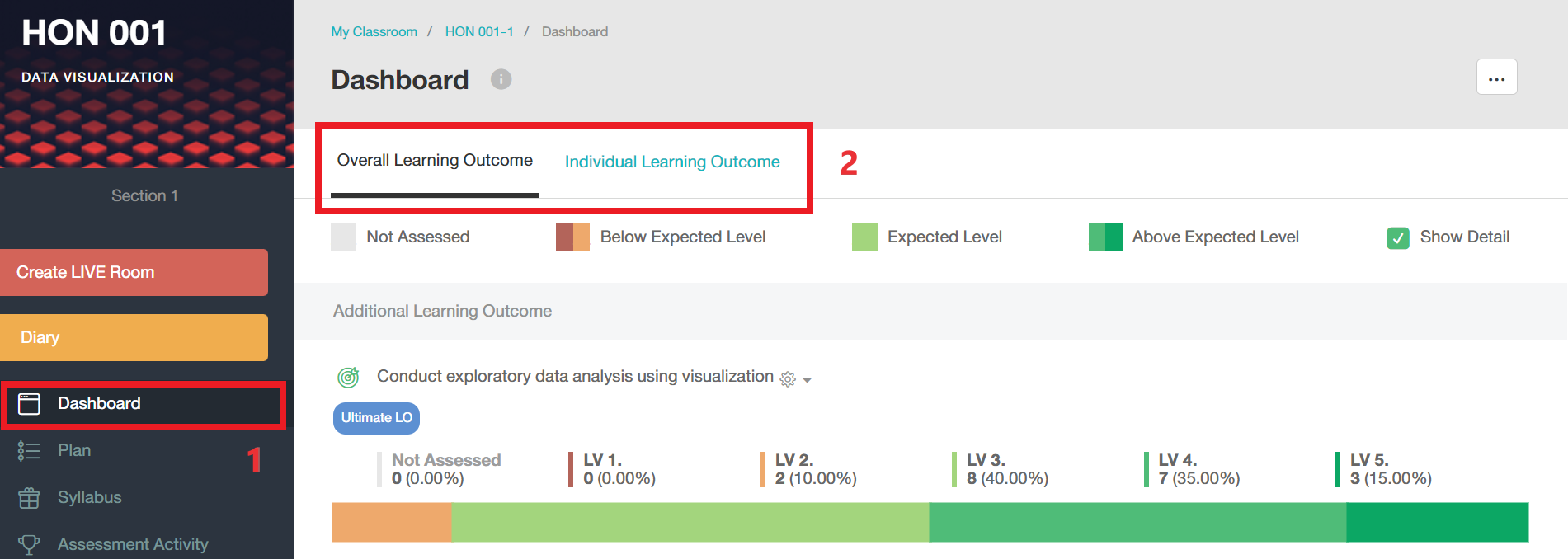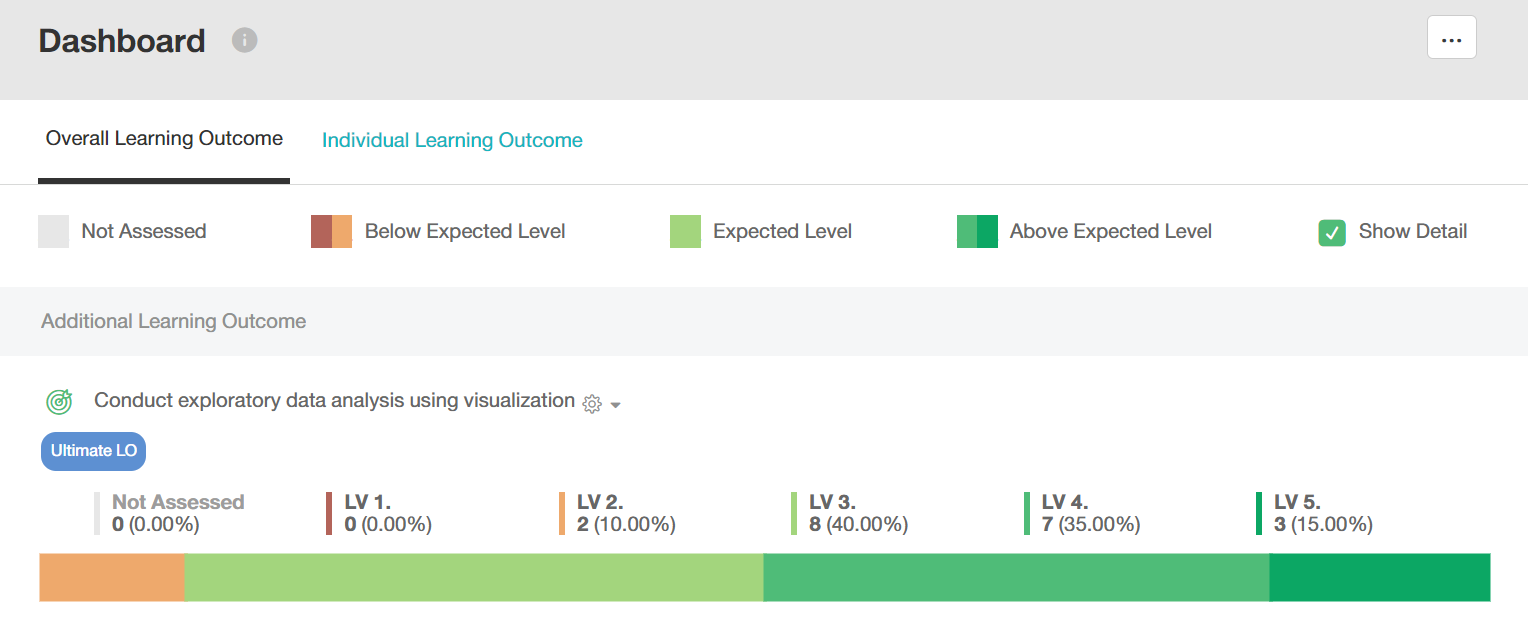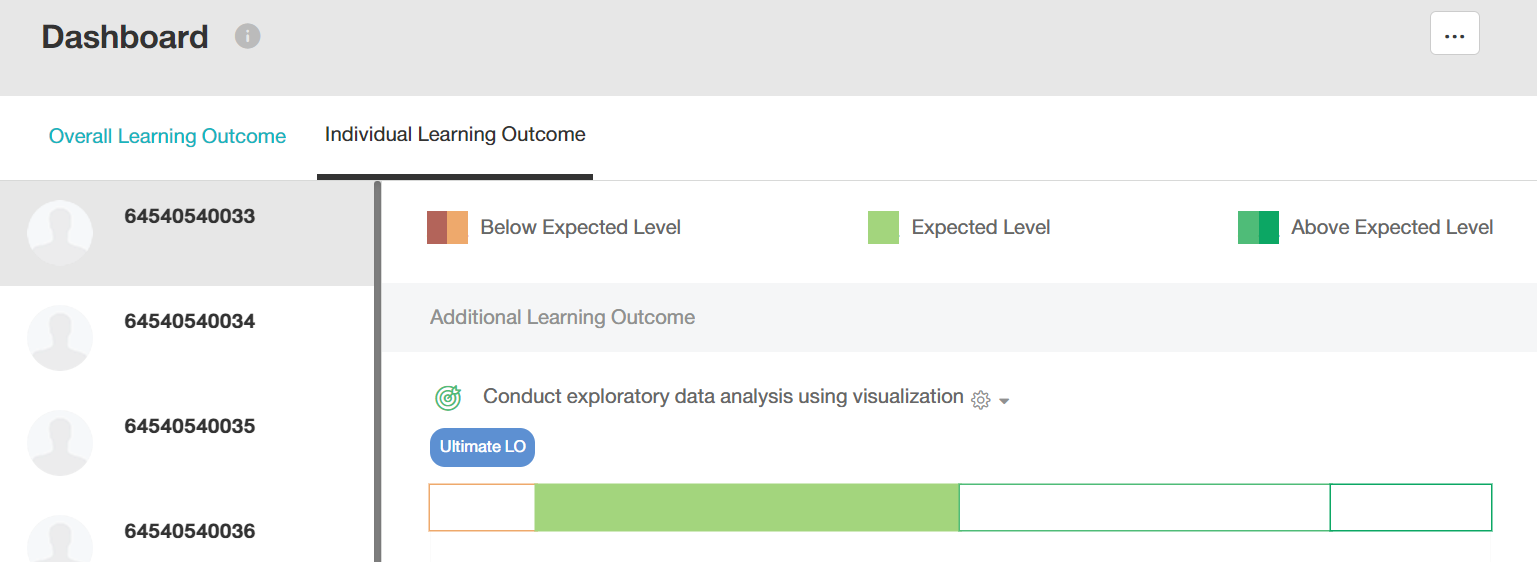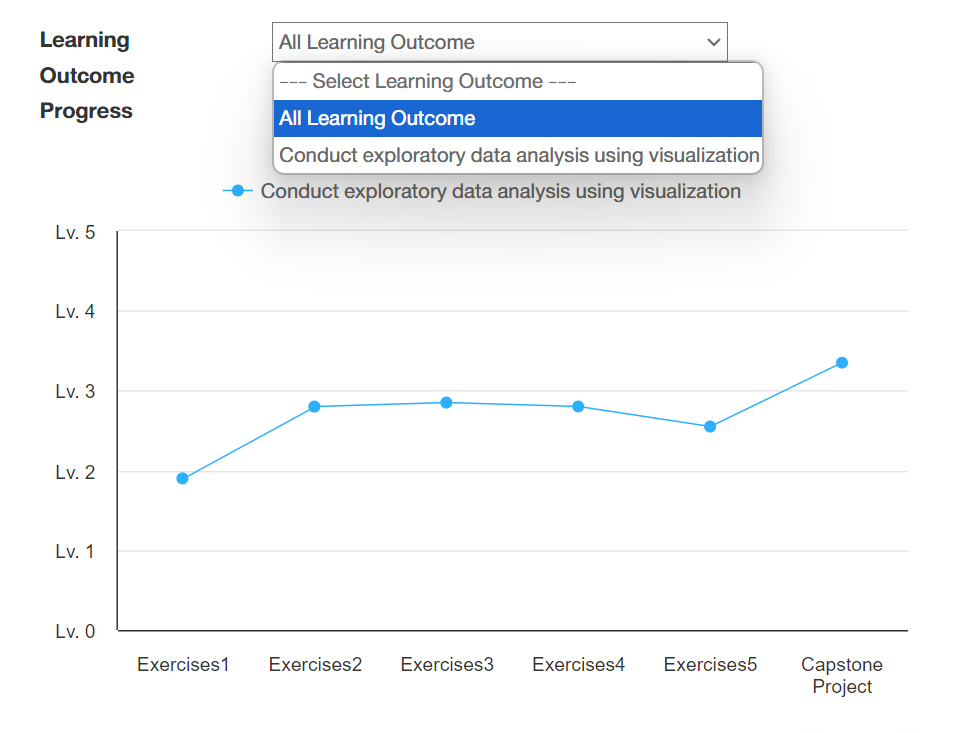# What Is Dashboard and How does It Work?

• Updated

#### What is Dashboard?

Dashboard is the feature that shows the summary of Learning Outcome which is aligned with Assessment Activity for the entire semester. The information can be seen as Overall Learning Outcome and Individual Learning Outcome. To access Dashboard, you may need to follow these steps;

1. Go to Dashboard
2. Select Overall Learning Outcome or Individual Learning Outcome to see the detail#### How does it work ?

There are 2 types of graph that shown in Dashboard, which are

• Bar graph
• Line graph
The bar graph
Figure 1 illustrates the competency of the students in the whole class by each Learning Outcome. The Learning Outcome is divided into 5 levels. If you want to see more in detail, mark at 'Show Detail', you will see the number of students who are at that level (It also shows how the per cent of the number of students at each level from the total number). This graph can tell the teachers how many students are at expected level and how many students need a support.Figure 1. Overall Learning Outcomes

Figure 2 illustrates an individual's competency for an outcome categorized into 5 levels as the graph shown in the whole class. Additionally, the individual graph will be portrayed as the students' competency at a level they can reach.Figure 2. Individual Learning Outcomes

The line graph
Figure 3 illustrates an Assessment Activity in which the students meet the most expected level of the outcomes. Teachers can see either overall outcomes or individual outcomes as selection. Additionally, teachers can filter to see each learning outcome as shown below.Figure 3. Learning Outcomes for activities in Learning Outcome
Related articles